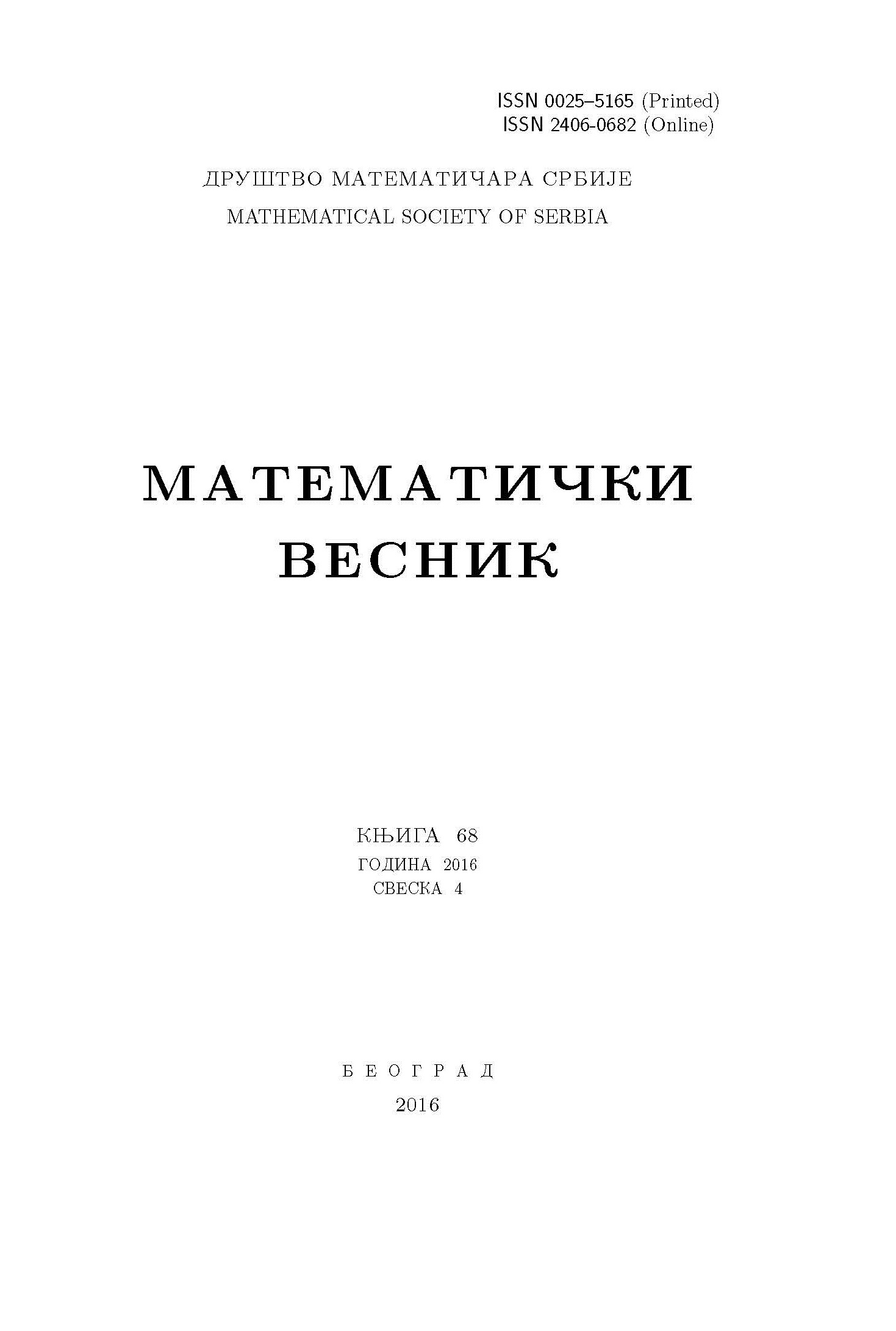﻿ Matematički Vesnik ﻿
 MATEMATIČKI VESNIK МАТЕМАТИЧКИ ВЕСНИКAPPROXIMATION SPACES VIA IDEALS AND GRILLS I. Ibedou, S. E. Abbas AbstractIn this paper, we use the notions of lower set $L_{R}(A)$ and the upper set $U_{R}(A)$ to define the interior operator ${\rm int}_{R}^{A}$ and the closure operator ${\rm cl}_{R}^{A}$ associated with a set $A$ in an approximation space $(X,R)$. These operators generate an approximation topological space different from the generated Nano topological space in $(X,R)$. Ideal approximation spaces $(X,R, \ell)$ based on an ideal $\ell$ joined to the approximation space $(X,R)$ are introduced as well. The approximation continuity and the ideal approximation continuity are defined. The lower separation axioms $T_{i}, i= 0,1,2$ are introduced in the approximation spaces and also in the ideal approximation spaces. Examples are given to explain the definitions. Connectedness in approximation spaces and ideal connectedness are introduced and the differences between them are explained. The interior and the closure operators are deduced using a grill ${\cal G}$ defined on $(X,R)$, yielding the same results.Keywords: Rough set; approximation space; approximation continuity; ideal approximation continuity; separation axioms; approximation connectedness; ideal approximation connectedness. MSC: 54A40, 54A05, 54A10, 03E20 Pages:  1$-$12

﻿